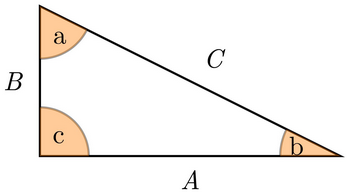# MTEL Mathematics (Middle School)(47): Practice & Study Guide Final Exam

Free Practice Test Instructions:

Choose your answer to the question and click 'Continue' to see how you did. Then click 'Next Question' to answer the next question. When you have completed the free practice test, click 'View Results' to see your results. Good luck!

#### Question 5 5. Given the right triangle in the figure below, what is the length of Side C if the lengths of Sides A and B are 24 inches and 32 inches, respectively?#### Question 12 12. Given the right triangle below, what is the value of the tangent function for angle b?